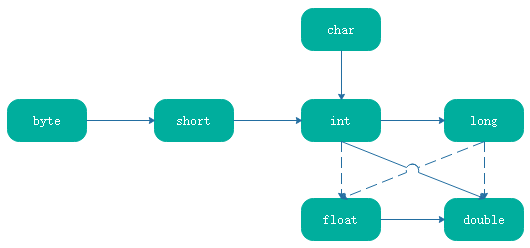• Java 教程
• Java 简介
• Java 基础
• Java 运算符
• Java 表达式
• Java 流程控制
• Java 数组
• Java 面向对象
• Java 集合类
• Java 异常处理
• Java 泛型
• Java 内置工具
• 参考手册

# 表达式数字类型转换```public class Demo {

public static void main(String[] args) {
int a = 123456789;
float b = a;
System.out.println("a=" + a);
System.out.println("b=" + b);
}

}```

```a=123456789
b=1.23456792E8```

• 如果两个操作数中有一个是 double 类型，另一个操作数需要转换为 double 类型

• 如果两个操作数中有一个是 float 类型，另一个操作数需要转换为 float 类型

• 如果两个操作数中有一个是 long 类型，另一个操作数需要转换为 long 类型

• 最后，两个操作数都将转换为 int 类型

```public class Demo {

public static void main(String[] args) {
int a = 1;
long b = 2L;
float c = 1.0f;
double d = 2.0D;
short e = 1;
byte f = 1;
// 如果两个操作数中有一个是 double 类型，另一个操作数需要转换为 double 类型
double r1 = a + d;
System.out.println("r1=" + r1);
// 如果两个操作数中有一个是 float 类型，另一个操作数需要转换为 float 类型
float r2 = a + c;
System.out.println("r2=" + r2);
// 如果两个操作数中有一个是 long 类型，另一个操作数需要转换为 long 类型
long r3 = a + b;
System.out.println("r3=" + r3);
// 最后，两个操作数都将转换为 int 类型
int r4 = a + e + f;
System.out.println("r4=" + r4);
}

}```

```r1=3.0
r2=2.0
r3=3
r4=3```

## 强制类型转换

`(目标数据类型)<表达式>`

```public class Demo {

public static void main(String[] args) {
int a = (int)3.14f;
System.out.println("a=" + a);

int b = (int)1890L;
System.out.println("b=" + b);

float c = (float)a + b;
System.out.println("c=" + c);

byte d = (byte)a;
System.out.println("d=" + d);
}

}```

```a=3
b=1890
c=1893.0
d=3```